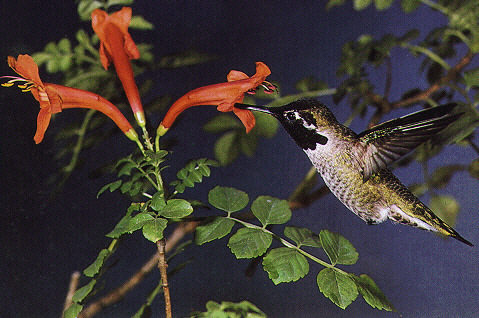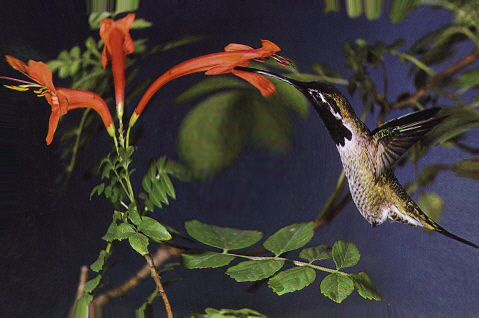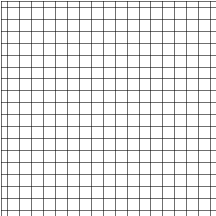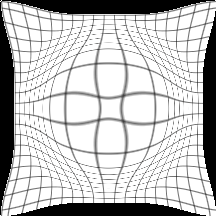←Select platform
In This Topic ▼

Summary
Wraps the image along its radius on a 3D surface shaped by Curve. This command is available in the Imaging Pro and above toolkits.
Syntax
C#
C++/CLI
Python
``public class FreeRadialBendCommand : RasterCommand ``
``public ref class FreeRadialBendCommand : public RasterCommand   ``
``class FreeRadialBendCommand(RasterCommand): ``
Remarks
• If the image has a region, the effect will be applied on the region only.
• For an example, the following figure shows an image before the effect is applied.The following figure shows the same image after the effect has been applied.• This command supports 12-bit and 16-bit grayscale and 48-bit and 64-bit color images. Support for 12-bit and 16-bit grayscale and 48-bit and 64-bit color images is available only in the Document/Medical toolkits.

• This command does not support signed data images.
• This command does not support 32-bit grayscale images.

Free Radial Bend Function - BeforeFree Radial Bend Function - AfterExample

Run the FreeRadialBendCommand on an image.

C#
````using Leadtools; `
`using Leadtools.Codecs; `
`using Leadtools.ImageProcessing.SpecialEffects; `
`using Leadtools.ImageProcessing.Effects; `
` `
` `
`public void FreeRadialBendCommandExample() `
`{ `
`   // Load an image `
`   RasterCodecs codecs = new RasterCodecs(); `
`   codecs.ThrowExceptionsOnInvalidImages = true; `
` `
`   RasterImage image = codecs.Load(Path.Combine(LEAD_VARS.ImagesDir, "sample5.cmp")); `
` `
`   // Prepare the command `
`   FreeRadialBendCommand command = new FreeRadialBendCommand(); `
`   LeadPoint[] UserPoint = new LeadPoint; `
`   LeadPoint CenterPoint = new LeadPoint(image.Width / 2, image.Height / 2); `
`   int[] Curve = new int; `
` `
`   //Apply the filter `
`   UserPoint.X = 0; `
`   UserPoint.Y = 0; `
`   UserPoint.X = 3; `
`   UserPoint.Y = 10; `
`   UserPoint.X = 9; `
`   UserPoint.Y = -10; `
`   UserPoint.X = 12; `
`   UserPoint.Y = 0; `
` `
`   EffectsUtilities.GetCurvePoints(Curve, UserPoint, CurvePointsType.Linear); `
`   for (int i = 0; i < Curve.Length; i++) `
`      Curve[i] /= 50; `
`   command.Flags = FreeRadialBendCommandFlags.Color | FreeRadialBendCommandFlags.Rotate; `
`   command.CenterPoint = CenterPoint; `
`   command.Scale = 200; `
`   command.FillColor = new RasterColor(255, 0, 0); `
`   command.Curve = Curve; `
`   command.Run(image); `
`   codecs.Save(image, Path.Combine(LEAD_VARS.ImagesDir, "Result.jpg"), RasterImageFormat.Jpeg, 24); `
` `
`} `
` `
`static class LEAD_VARS `
`{ `
`   public const string ImagesDir = @"C:\LEADTOOLS22\Resources\Images"; `
`} ````
Requirements
Help Version 22.0.2023.3.31